# Stoichiometry, Gas StoichiometryPage 1

#### WATCH ALL SLIDES

Slide 1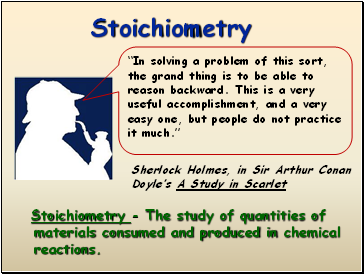## Stoichiometry

Sherlock Holmes, in Sir Arthur Conan Doyle’s A Study in Scarlet

“In solving a problem of this sort, the grand thing is to be able to reason backward. This is a very useful accomplishment, and a very easy one, but people do not practice it much.”

Stoichiometry - The study of quantities of materials consumed and produced in chemical reactions.

Slide 2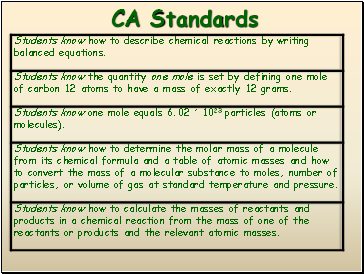CA Standards

Slide 3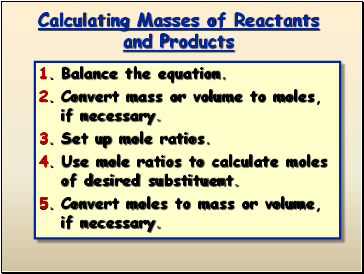## Calculating Masses of Reactants and Products

Balance the equation.

Convert mass or volume to moles, if necessary.

Set up mole ratios.

Use mole ratios to calculate moles of desired substituent.

Convert moles to mass or volume, if necessary.

Slide 4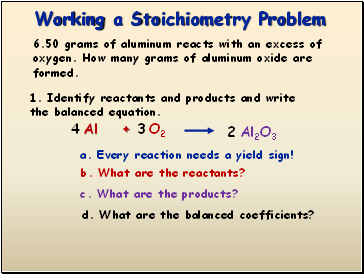## Working a Stoichiometry Problem

6.50 grams of aluminum reacts with an excess of oxygen. How many grams of aluminum oxide are formed.

1. Identify reactants and products and write the balanced equation.

Al

+

O2

Al2O3

b. What are the reactants?

a. Every reaction needs a yield sign!

c. What are the products?

d. What are the balanced coefficients?

4

3

2

Slide 5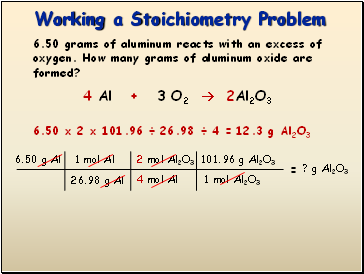Working a Stoichiometry Problem

6.50 grams of aluminum reacts with an excess of oxygen. How many grams of aluminum oxide are formed?

4 Al + 3 O2  2Al2O3

=

6.50 g Al

? g Al2O3

1 mol Al

26.98 g Al

4 mol Al

2 mol Al2O3

1 mol Al2O3

101.96 g Al2O3

6.50 x 2 x 101.96 ÷ 26.98 ÷ 4 =

12.3 g Al2O3

Slide 6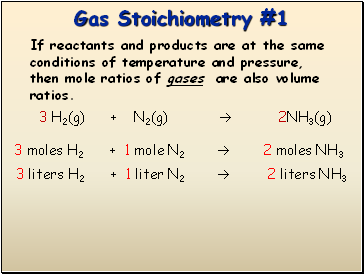## Gas Stoichiometry

#1

If reactants and products are at the same conditions of temperature and pressure, then mole ratios of gases are also volume ratios.

3 H2(g) + N2(g)  2NH3(g)

3 moles H2 + 1 mole N2  2 moles NH3

3 liters H2 + 1 liter N2  2 liters NH3

Slide 7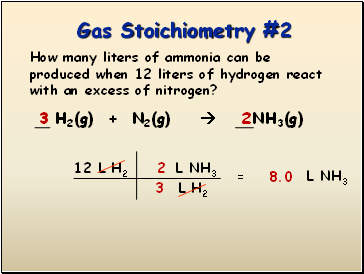Gas Stoichiometry #2

How many liters of ammonia can be produced when 12 liters of hydrogen react with an excess of nitrogen?

3 H2(g) + N2(g)  2NH3(g)

12 L H2

L H2

= L NH3

L NH3

3

2

8.0

Slide 8Gas Stoichiometry #3

How many liters of oxygen gas, at STP, can be collected from the complete decomposition of 50.0 grams of potassium chlorate?

2 KClO3(s)  2 KCl(s) + 3 O2(g)

50.0 g KClO3

Go to page:
1  2